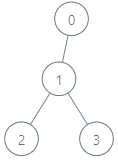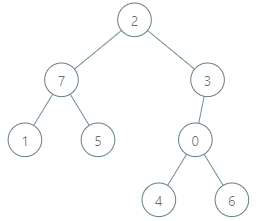##### Welcome to Subscribe On Youtube

Formatted question description: https://leetcode.ca/all/1938.html

# 1938. Maximum Genetic Difference Query

Hard

## Description

There is a rooted tree consisting of n nodes numbered 0 to n - 1. Each node’s number denotes its unique genetic value (i.e. the genetic value of node x is x). The genetic difference between two genetic values is defined as the bitwise-XOR of their values. You are given the integer array parents, where parents[i] is the parent for node i. If node x is the root of the tree, then parents[x] == -1.

You are also given the array queries where queries[i] = [node_i, val_i]. For each query i, find the maximum genetic difference between val_i and p_i, where p_i is the genetic value of any node that is on the path between node_i and the root (including node_i and the root). More formally, you want to maximize val_i XOR p_i.

Return an array ans where ans[i] is the answer to the i-th query.

Example 1:Input: parents = [-1,0,1,1], queries = [0,2,3,2,2,5]

Output: [2,3,7]

Explanation: The queries are processed as follows:

Example 2:Input: parents = [3,7,-1,2,0,7,0,2], queries = [4,6,1,15,0,5]

Output: [6,14,7]

Explanation: The queries are processed as follows:

Constraints:

• 2 <= parents.length <= 10^5
• 0 <= parents[i] <= parents.length - 1 for every node i that is not the root.
• parents[root] == -1
• 1 <= queries.length <= 3 * 10^4
• 0 <= node_i <= parents.length - 1
• 0 <= val_i <= 2 * 10^5

## Solution

Create a trie that stores the numbers in nums. Each node in the trie has two children that represent bits 0 and 1, respectively. For each number, create the trie from the highest bit to the lowest bit, where all the numbers have at most 18 bits. For each node in the trie, maintain the number of times that the node is passed by.

Then do depth first search on the trie starting from the root to obtain the query results.

Finally, return the query results.

• class Solution {
static final int MAXD = 17;

public int[] maxGeneticDifference(int[] parents, int[][] queries) {
int n = parents.length;
List<List<Integer>> edges = new ArrayList<List<Integer>>();
for (int i = 0; i < n; i++)
int rootIndex = -1;
for (int i = 0; i < n; i++) {
if (parents[i] == -1)
rootIndex = i;
else
}
List<List<int[]>> stored = new ArrayList<List<int[]>>();
for (int i = 0; i < n; i++)
int queriesCount = queries.length;
int[] ans = new int[queriesCount];
for (int i = 0; i < queriesCount; i++)
TrieNode root = new TrieNode();
depthFirstSearch(ans, root, rootIndex, edges, stored);
return ans;
}

public void insert(TrieNode root, int x) {
TrieNode curr = root;
for (int i = MAXD; i >= 0; i--) {
if ((x & (1 << i)) != 0) {
if (curr.children == null)
curr.children = new TrieNode();
curr = curr.children;
} else {
if (curr.children == null)
curr.children = new TrieNode();
curr = curr.children;
}
curr.count++;
}
}

public int query(TrieNode root, int x) {
int queryMax = 0;
TrieNode curr = root;
for (int i = MAXD; i >= 0; i--) {
if ((x & (1 << i)) != 0) {
if (curr.children != null && curr.children.count > 0) {
queryMax |= 1 << i;
curr = curr.children;
} else
curr = curr.children;
} else {
if (curr.children != null && curr.children.count > 0) {
queryMax |= 1 << i;
curr = curr.children;
} else
curr = curr.children;
}
}
return queryMax;
}

public void erase(TrieNode root, int x) {
TrieNode curr = root;
for (int i = MAXD; i >= 0; i--) {
if ((x & (1 << i)) != 0)
curr = curr.children;
else
curr = curr.children;
curr.count--;
}
}

public void depthFirstSearch(int[] ans, TrieNode root, int node, List<List<Integer>> edges, List<List<int[]>> stored) {
insert(root, node);
List<int[]> list = stored.get(node);
for (int[] pair : list) {
int index = pair, num = pair;
ans[index] = query(root, num);
}
List<Integer> nextNodes = edges.get(node);
for (int nextNode : nextNodes)
depthFirstSearch(ans, root, nextNode, edges, stored);
erase(root, node);
}
}

class TrieNode {
int count;
TrieNode[] children;

public TrieNode() {
children = new TrieNode;
count = 0;
}
}

• // OJ: https://leetcode.com/problems/maximum-genetic-difference-query/
// Time: O(P + Q)
// Space: O(P)
struct TrieNode {
TrieNode *next = {};
int cnt = 0;
};
class Solution {
public:
vector<int> maxGeneticDifference(vector<int>& P, vector<vector<int>>& Q) {
int N = P.size(), M = Q.size(), rootIndex;
vector<vector<int>> G(N);
for (int i = 0; i < N; ++i) {
if (P[i] != -1) G[P[i]].push_back(i);
else rootIndex = i;
}
unordered_map<int, vector<int>> m;
for (int i = 0; i < M; ++i) m[Q[i]].push_back(i);
TrieNode root;
auto getAnswer = [&](TrieNode *node, int q) {
int ans = 0;
for (int i = 31; i >= 0; --i) {
int b = q >> i & 1;
if (node->next[1 - b] && node->next[1 - b]->cnt) {
node = node->next[1 - b];
ans |= 1 << i;
} else node = node->next[b];
}
return ans;
};
vector<int> ans(M);
function<void(int)> dfs = [&](int u) {
auto node = &root;
for (int i = 31; i >= 0; --i) {
int b = u >> i & 1;
if (!node->next[b]) node->next[b] = new TrieNode();
node = node->next[b];
node->cnt++;
}
if (m.count(u)) {
for (int index : m[u]) ans[index] = getAnswer(&root, Q[index]);
}
for (int v : G[u]) dfs(v);
node = &root;
for (int i = 31; i >= 0; --i) {
int b = u >> i & 1;
node = node->next[b];
node->cnt--;
}
};
dfs(rootIndex);
return ans;
}
};